﻿ Iron Loss by Input Current with Arbitrary Waveform (Triangle Wave)Examples | Product | Murata Software Co., Ltd.Example12Iron Loss by Input Current with Arbitrary Waveform (Triangle Wave)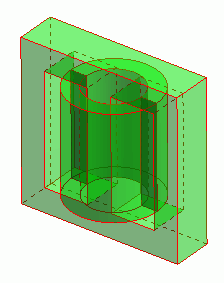General

• The iron loss is calculated when the arbitrary-waveform current is input to the coil wound on a core. In this example, the waveform is triangle wave.
A square wave can be analyzed in the same way.

• To calculate the iron loss with the arbitrary waveform, [Frequency Analysis 8FFT)] is used as a calculation method.

• The core loss, the vectors of the magnetic field and the magnetic flux density are solved.

• Unless specified in the list below, the default conditions will be applied.

Analysis Space

 Item Setting Analysis Space 3D Model Unit mm

Analysis Conditions

 Item Setting Solver Magnetic Analysis [Luvens] Analysis Type Transient Analysis Options None

The Transient Analysis tab is set up as follows.

The input is 50 [kHz]. In order to reproduce the triangle waveform well, and also in order to take into account the frequency component high enough to calculate the iron loss by FFT,
the timestep is one period of 50 [kHz] divided by 24.

Tab

Setting Item

Setting

Transient Analysis

Timestep

Automatic

Table

 Number Calculation steps Output steps Timestep [s] 1 24 1 1.0/(24*50e3)

Model

A coil is wound on a core.

The model is defined by the core (Core) and the loop coil (Coil) are defined.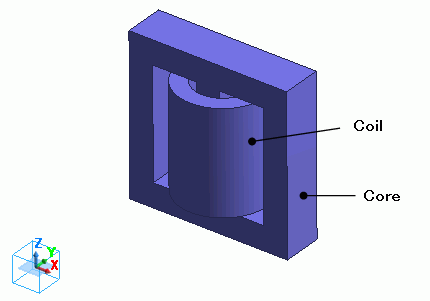Body Attributes and Materials

 Body Number/Type Body Attribute Name Material Name 5/Solid Coil 008_Cu * 6/Solid Core MB3_23deg_JFE Ferrite *

* Available from the material DB

The material properties are set up as follows:

[MB3_23deg_JFE Ferrite] is used for the core.

The values for the B-H curve and the iron loss table are taken from the database. The iron loss calculation type is manually changed to [Frequency Analysis (FFT)] from [Amplitude].
The iron loss calculation by the iron loss table is executed by comparing the the amplitude of the frequency and the magnetic flux density with the table.
if the sine wave is not used, the harmonic component of the iron loss cannot be taken into account unless the frequency analysis (FFT) is performed.

See Permeability tab for detail.

 Material Name Tab Setting MB3_23deg_JFE Ferrite Iron Loss Calculation Method: Frequency Analysis (FFT) Fundamental frequency: Specify frequency/50[kHz)

Body attribute is set up as follows to apply current to the loop coil.

The current is a triangle wave with frequency of 50kHz and amplitude of 1A.

Body Attribute Name

Tab

Setting

Coil

Current

Waveform: Arbitrary waveform

[Time-Current] Table

 Time [s] Current [A] 0 0 1/4/50e3 1.0 2/4/50e3 0 3/4/50e3 -1.0 4/4/50e3 0

Current: 0.1[A]

Frequency: 50×103[Hz]

Turns: 100[Turns]

Direction: Loop Coil/Magnetic Field Direction

Direction Vector of Magnetic Field: X=0, Y=0, Z=1

Enter the values covering one phase in the [Time-Current] table and the current will repeat automatically.

By pressing the graph button of the [Time-Current] table, the repetition can be seen as shown below.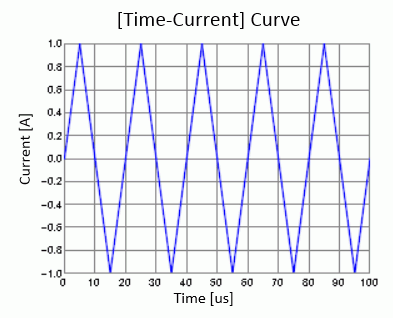No setting.

Results

To see the iron loss, go to the [Results] tab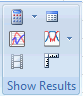and click [Table]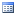.

The results are shown on the [Loss [W] (referring to value over 1 period from the final step)] tab as below.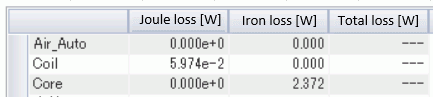Each frequency component is output on the [Frequency component (FFT) of iron loss [W]] tab.

Below is the results plotted in a graph.

AS the input current is a triangle wave, harmonic components can be seen.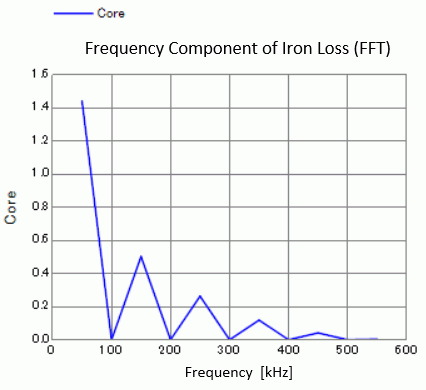• See [Loss Calculation in the Magnetic Analysis] for details of the loss.

The vectors of the magnetic field at timestep 1 are shown below.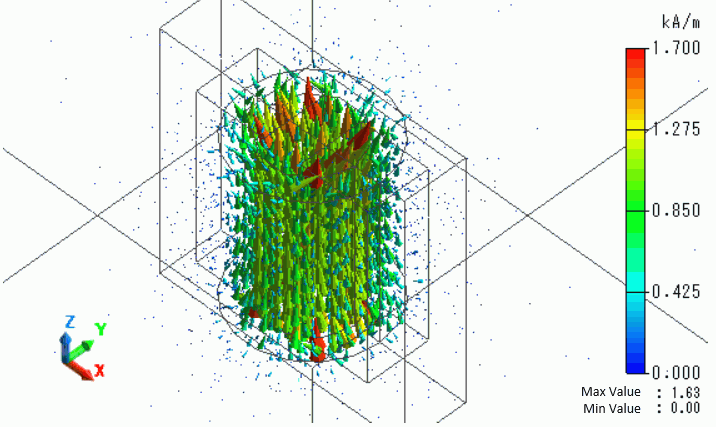The vectors of the magnetic flux density at timestep 1 are shown below.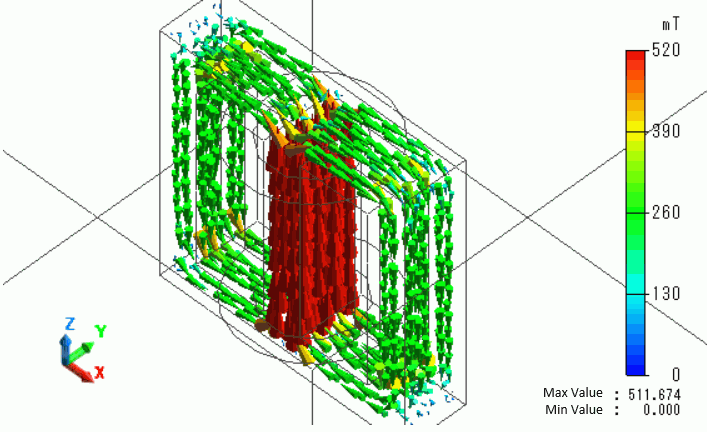The iron loss density is shown below.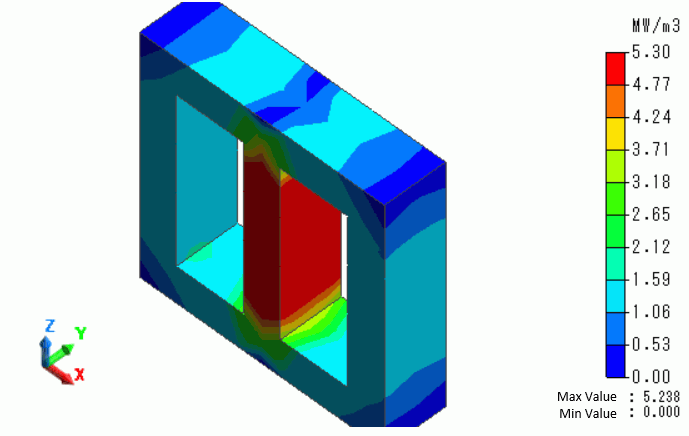﻿